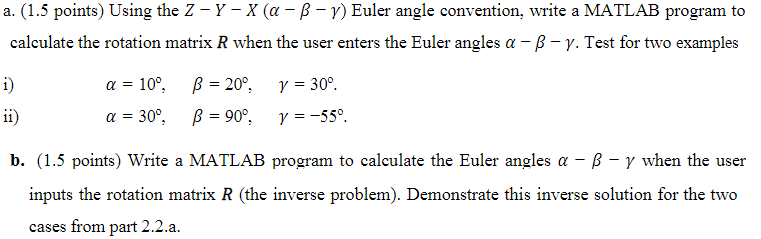Home / Expert Answers / Mechanical Engineering / a-1-5-points-using-the-zyx-euler-angle-convention-write-a-matlab-program-to-pa666

# (Solved): a. (1.5 points) Using the ZYX() Euler angle convention, write a MATLAB program to ...a. (1.5 points) Using the Euler angle convention, write a MATLAB program to calculate the rotation matrix when the user enters the Euler angles . Test for two examples i) b. (1.5 points) Write a MATLAB program to calculate the Euler angles when the user inputs the rotation matrix (the inverse problem). Demonstrate this inverse solution for the two cases from part 2.2.a.

We have an Answer from Expert